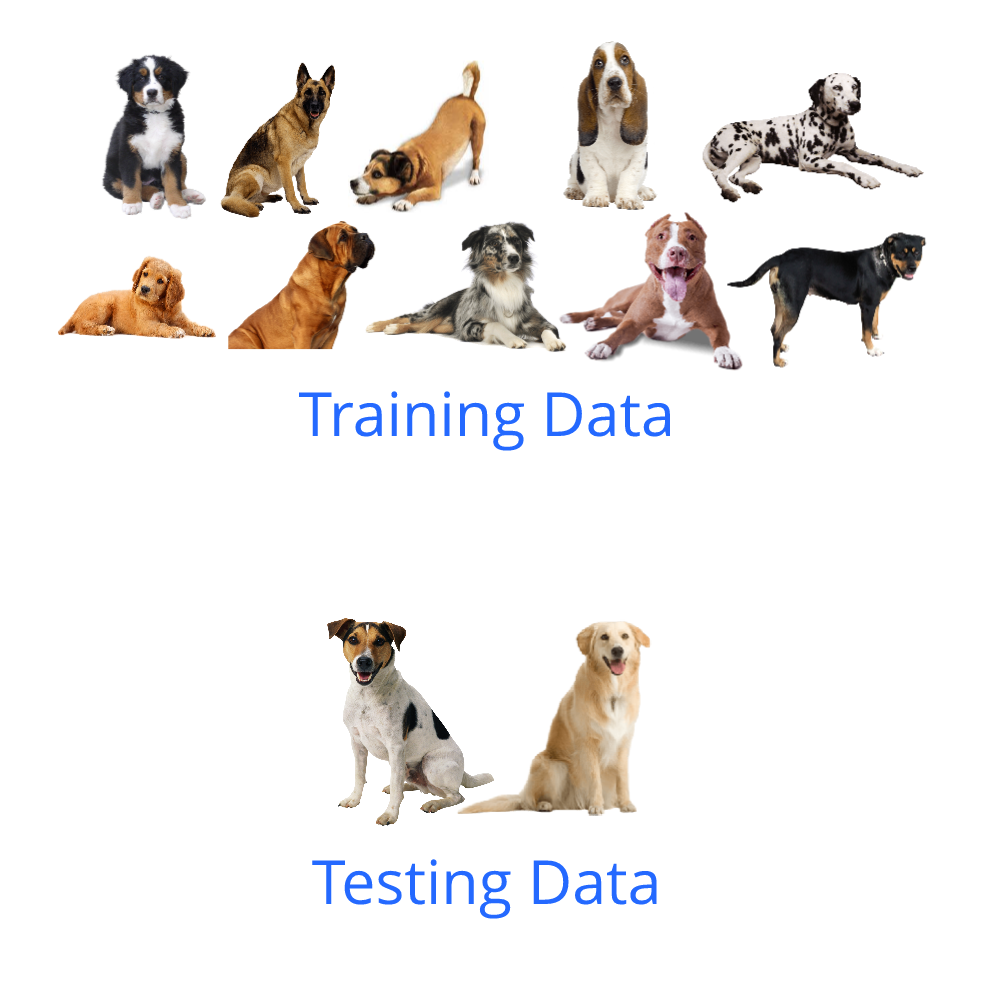# Generalization in Neural Networks

Source: Deep Learning on Medium# Generalization in Neural Networks

Whenever we train our own Neural Networks, we need to take care of something called the generalization of the Neural Network. This essentially means how good our model is at learning from the given data and applying the learnt information elsewhere.

When training a neural network, there’s going to be some data which the Neural Network trains on, and there’s going to be some data reserved for checking the performance of the Neural Network. If the Neural Network performs well on the data which it has not trained on, we can say it has generalized well on the given data. Let’s understand this with an example.

Suppose we are training a neural network which should tell us if a given image has a dog or not. Let’s assume we have several pictures of dogs, each dog belonging to a certain breed, and there are 12 total breeds within those pictures. I’m going to keep all the images of 10 breeds of dogs for training and the remaining images of the 2 breeds will be kept aside for now.

Now before going to the Deep Learning side of things, let’s look at this from a human perspective. Let’s consider a human being who has never seen a dog in their entire life (just for the sake of an example). Now we will show this human the 10 breeds of dogs and tell them that these are dogs. After this, if we show them the other 2 breeds, will they be able to tell that they are also dogs? Well hopefully they should, 10 breeds should be enough to understand and identify the unique features of a dog. This concept of learning from some data and correctly applying the gained knowledge on other data is called generalization.

Coming back to deep learning, our aim is to make the neural network learn as effectively from the given data as possible. If we successfully make the neural network understand that the other 2 breeds are also dogs, then we have trained a very general neural network and it will perform really well in the real world.

This is actually easier said than done and training a general neural network is one of the most frustrating tasks of a deep learning practitioner. This is because of a phenomenon in neural networks called overfitting. If the neural network trains on the 10 breeds of dogs and refuses to classify the other 2 breeds of dogs as dogs, then this neural network has overfit on the training data. What this means is that the neural network has memorized those 10 breeds of dogs and considers only them to be dogs. Due to this, it fails to form a general understanding of what dogs look like. Combating this issue while training Neural Networks is what we are going to be looking at in this article.

Now we don’t actually have the liberty to divide all our data on a basis like breed, instead we will simply split all the data. One part of the data, usually the bigger part (around 80–90%), will be used for training the model, and the rest will be used to test it. Our objective is to make sure that the performance on the testing data is around the same as the performance on the training data. We use metrics like loss and accuracy to measure this performance.

There are certain aspects of neural networks which we can control in order to prevent overfitting. Let’s go through them one by one. The first thing is number of parameters.

## Number of Parameters

In a neural network, the number of parameters essentially means the number of weights. This is going to be directly proportional to the number of layers and the number of neurons in each layer. The relationship between number of parameters and overfitting is as follows: the more the parameters, the more the chance of overfitting. I’ll explain why.

We need to define our problem in terms of complexity. A very complex dataset would require a very complex function to successfully understand and represent it. Mathematically speaking, we can somewhat associate complexity with non linearity. Let’s recall the neural network formula.